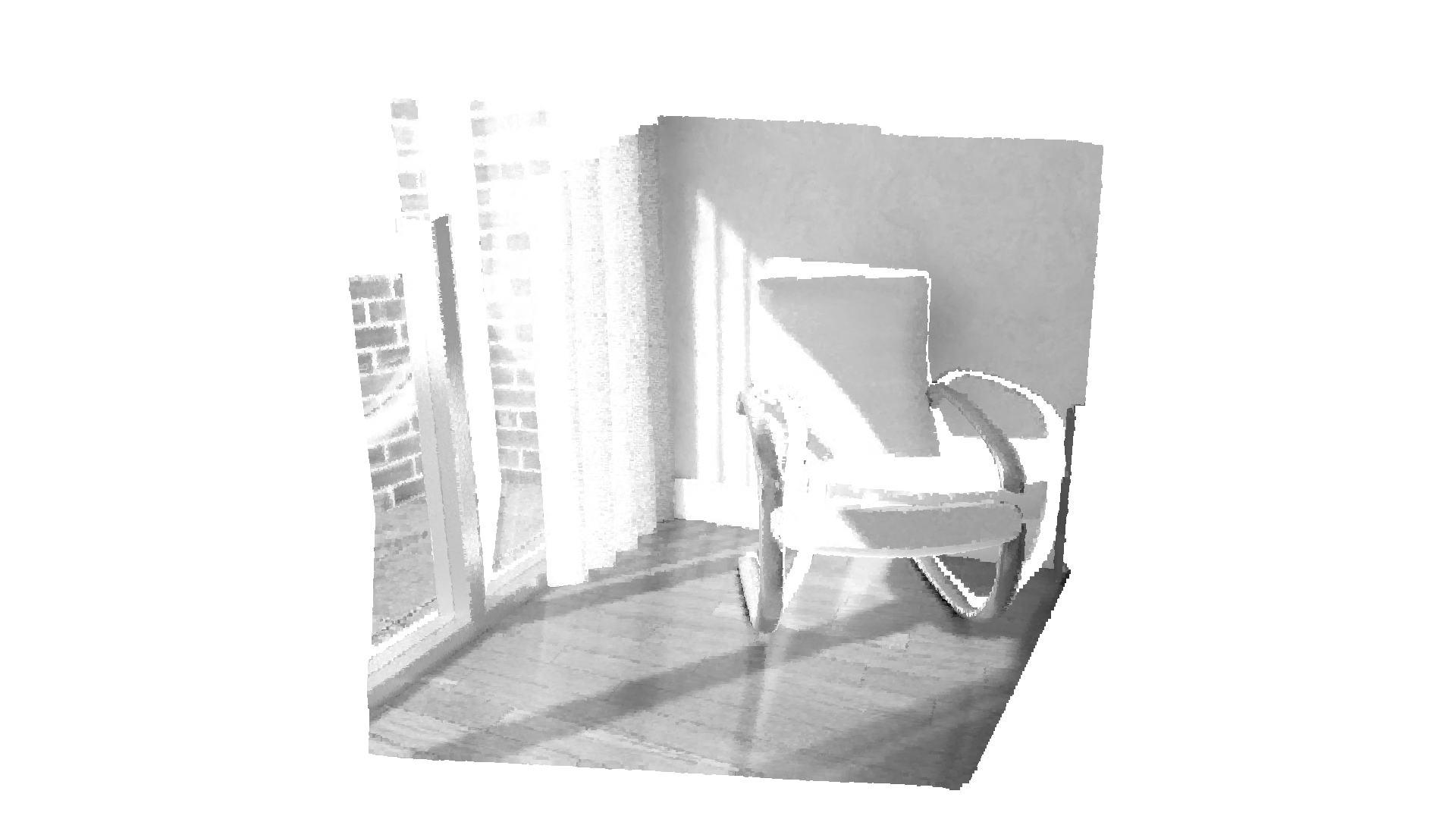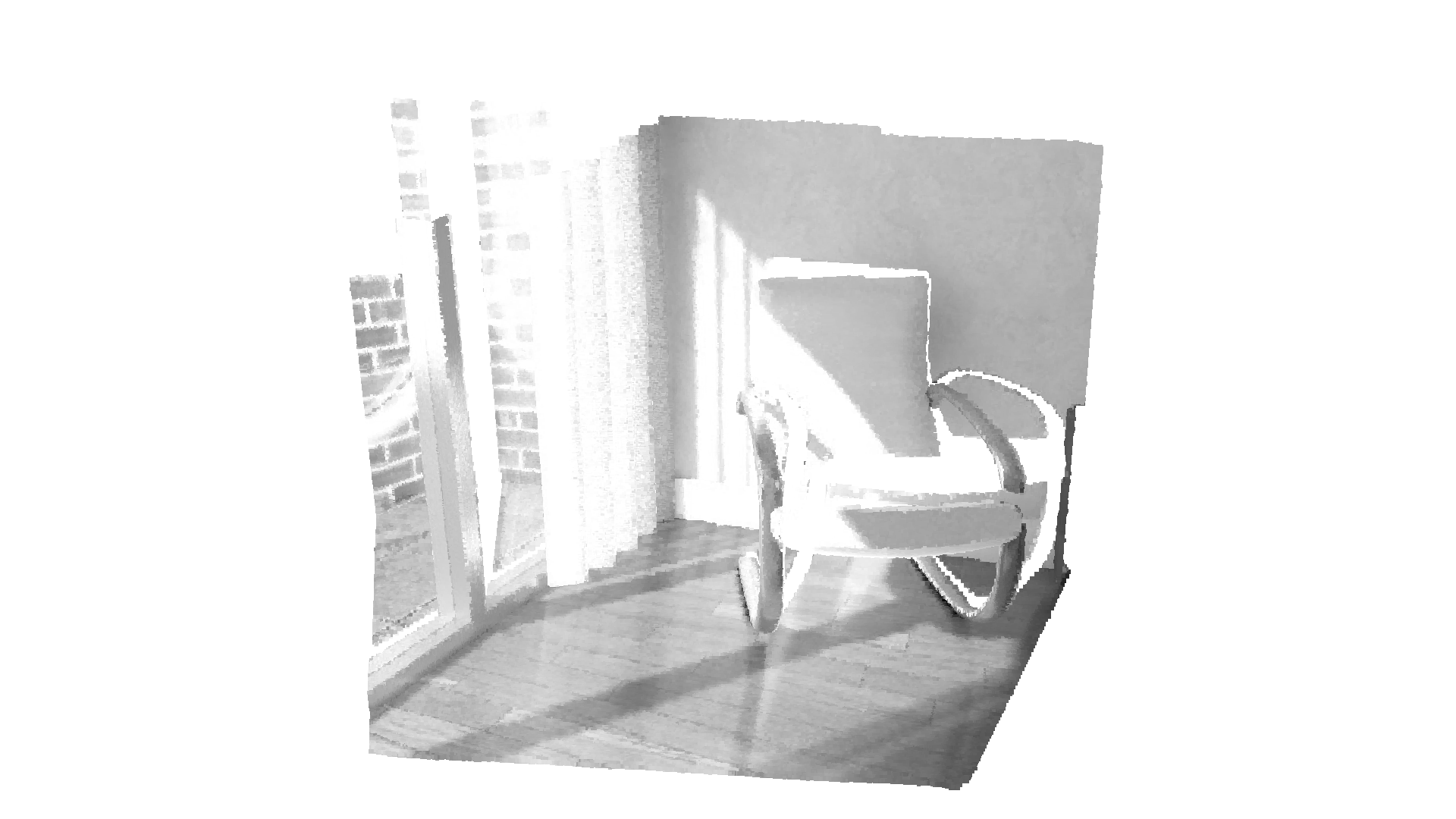# RGBD Odometry¶

An RGBD odometry finds the camera movement between two consecutive RGBD image pairs. The input are two instances of RGBDImage. The output is the motion in the form of a rigid body transformation. Open3D implements the method of [Steinbrucker2011] and [Park2017].

We first read the camera intrinsic matrix from a json file.

:

redwood_rgbd = o3d.data.SampleRedwoodRGBDImages()

redwood_rgbd.camera_intrinsic_path)
print(pinhole_camera_intrinsic.intrinsic_matrix)

[[525.    0.  319.5]
[  0.  525.  239.5]
[  0.    0.    1. ]]


Note:

Lots of small data structures in Open3D can be read from / written into json files. This includes camera intrinsics, camera trajectory, pose graph, etc.

This code block reads two pairs of RGBD images in the Redwood format. We refer to Redwood dataset for a comprehensive explanation.

:

source_color = o3d.io.read_image(redwood_rgbd.color_paths)
source_rgbd_image = o3d.geometry.RGBDImage.create_from_color_and_depth(
source_color, source_depth)
target_rgbd_image = o3d.geometry.RGBDImage.create_from_color_and_depth(
target_color, target_depth)
target_pcd = o3d.geometry.PointCloud.create_from_rgbd_image(
target_rgbd_image, pinhole_camera_intrinsic)


Note:

Open3D assumes the color image and depth image are synchronized and registered in the same coordinate frame. This can usually be done by turning on both the synchronization and registration features in the RGBD camera settings.

## Compute odometry from two RGBD image pairs¶

:

option = o3d.pipelines.odometry.OdometryOption()
odo_init = np.identity(4)
print(option)

[success_color_term, trans_color_term,
info] = o3d.pipelines.odometry.compute_rgbd_odometry(
source_rgbd_image, target_rgbd_image, pinhole_camera_intrinsic, odo_init,
o3d.pipelines.odometry.RGBDOdometryJacobianFromColorTerm(), option)
[success_hybrid_term, trans_hybrid_term,
info] = o3d.pipelines.odometry.compute_rgbd_odometry(
source_rgbd_image, target_rgbd_image, pinhole_camera_intrinsic, odo_init,
o3d.pipelines.odometry.RGBDOdometryJacobianFromHybridTerm(), option)

OdometryOption class.
iteration_number_per_pyramid_level = [ 20, 10, 5, ]
depth_diff_max = 0.030000
depth_min = 0.000000
depth_max = 4.000000


This code block calls two different RGBD odometry methods. The first one is from [Steinbrucker2011]. It minimizes photo consistency of aligned images. The second one is from [Park2017]. In addition to photo consistency, it implements constraint for geometry. Both functions run in similar speed, but [Park2017] is more accurate in our test on benchmark datasets and is thus the recommended method.

Several parameters in OdometryOption():

• minimum_correspondence_ratio: After alignment, measure the overlapping ratio of two RGBD images. If overlapping region of two RGBD image is smaller than specified ratio, the odometry module regards that this is a failure case.

• depth_diff_max: In depth image domain, if two aligned pixels have a depth difference less than specified value, they are considered as a correspondence. Larger value induce more aggressive search, but it is prone to unstable result.

• depth_min and depth_max: Pixels that has smaller or larger than specified depth values are ignored.

## Visualize RGBD image pairs¶

The RGBD image pairs are converted into point clouds and rendered together. Note that the point cloud representing the first (source) RGBD image is transformed with the transformation estimated by the odometry. After this transformation, both point clouds are aligned.

:

if success_color_term:
print("Using RGB-D Odometry")
print(trans_color_term)
source_pcd_color_term = o3d.geometry.PointCloud.create_from_rgbd_image(
source_rgbd_image, pinhole_camera_intrinsic)
source_pcd_color_term.transform(trans_color_term)
o3d.visualization.draw_geometries([target_pcd, source_pcd_color_term],
zoom=0.48,
front=[0.0999, -0.1787, -0.9788],
lookat=[0.0345, -0.0937, 1.8033],
up=[-0.0067, -0.9838, 0.1790])
if success_hybrid_term:
print("Using Hybrid RGB-D Odometry")
print(trans_hybrid_term)
source_pcd_hybrid_term = o3d.geometry.PointCloud.create_from_rgbd_image(
source_rgbd_image, pinhole_camera_intrinsic)
source_pcd_hybrid_term.transform(trans_hybrid_term)
o3d.visualization.draw_geometries([target_pcd, source_pcd_hybrid_term],
zoom=0.48,
front=[0.0999, -0.1787, -0.9788],
lookat=[0.0345, -0.0937, 1.8033],
up=[-0.0067, -0.9838, 0.1790])

Using RGB-D Odometry
[[ 9.99988286e-01 -7.53983409e-05 -4.83963172e-03  2.74054550e-04]
[ 1.83909052e-05  9.99930634e-01 -1.17782559e-02  2.29634918e-02]
[ 4.84018408e-03  1.17780289e-02  9.99918922e-01  6.02121265e-04]
[ 0.00000000e+00  0.00000000e+00  0.00000000e+00  1.00000000e+00]]Using Hybrid RGB-D Odometry
[[ 9.99992973e-01 -2.51084541e-04 -3.74035273e-03 -1.07049775e-03]
[ 2.07046059e-04  9.99930714e-01 -1.17696227e-02  2.32280983e-02]
[ 3.74304875e-03  1.17687656e-02  9.99923740e-01  1.40592054e-03]
[ 0.00000000e+00  0.00000000e+00  0.00000000e+00  1.00000000e+00]]